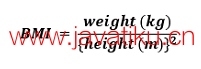# Python教程-使用Python创建BMI计算器## 理解身体质量指数（BMI）

BMI，即身体质量指数，是根据个体的质量和身高来衡量相对体重的一种方法。通常，我们使用身体质量指数来根据个人的身高体重对人们进行分类。这些分类包括体重不足，健康，超重，甚至肥胖。此外，各国还采用BMI来促进健康饮食。

## 理解BMI计算器的工作原理

BMI计算器接受个体的体重和身高，并计算该人的身体质量指数（BMI）。

1低于18.5体重不足
218.5 - 24.9正常
325.0 - 29.9超重
430.0 及以上肥胖

## 使用Python创建BMI计算器

``````# asking for input from the users
the_height = float(input("Enter the height in cm: "))
the_weight = float(input("Enter the weight in kg: ")) ````````````# defining a function for BMI
the_BMI = the_weight / (the_height/100)**2  ``````

``````# printing the BMI
print("Your Body Mass Index is", the_BMI)  ``````

``````# using the if-elif-else conditions
if the_BMI <= 18.5:
print("Oops! You are underweight.")
elif the_BMI <= 24.9:
print("Awesome! You are healthy.")
elif the_BMI <= 29.9:
the_print("Eee! You are overweight.")
else:
print("Seesh! You are obese.")  ``````

1. 如果BMI小于或等于18.5，则程序返回体重不足的条件。
2. 如果BMI小于或等于24.9，则程序返回健康的条件。
3. 如果BMI小于或等于29.9，则程序返回超重的条件。
4. 如果上述条件都不为，则程序返回肥胖的条件。

## 源代码

``````# asking for input from the users
the_height = float(input("Enter the height in cm: "))
the_weight = float(input("Enter the weight in kg: "))
# defining a function for BMI
the_BMI = the_weight / (the_height/100)**2
# printing the BMI
print("Your Body Mass Index is", the_BMI)
# using the if-elif-else conditions
if the_BMI <= 18.5:
print("Oops! You are underweight.")
elif the_BMI <= 24.9:
print("Awesome! You are healthy.")
elif the_BMI <= 29.9:
the_print("Eee! You are over weight.")
else:
print("Seesh! You are obese.")  ``````

``````Enter the height in cm: 160
Enter the weight in kg: 61
Your Body Mass Index is 23.828124999999996
Awesome! You are healthy.``````